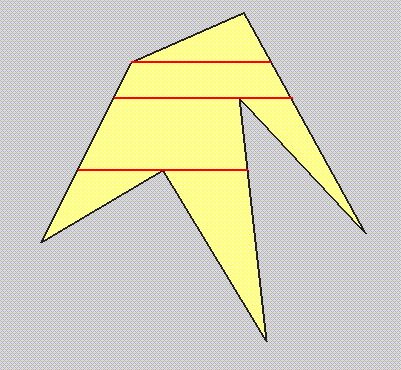# Trapezoidal Decomposition

Suppose we have a simple polygon with vertices p0, p1, ..., pn-1. From each vertex, cast a horizontal line to the left and also to the right, each of which terminates when it intersects another polygon edge (or vertex). Some of the lines will be outside the polygon (for example, based on the even-odd test) and these lines should be discarded.

An example is shown below, where the polygon is outlined in black, and the edges of this polygon together with the new (red) horizontal lines form a number of trapezia (we are considering a triangle as a degenerate trapezium).## Applications

There are several applications of such trapezoidal decompositions. One is that since it is fast and easy to fill a trapezium, a complex shape can be deconstructed into a set of simpler ones, each of which can be scan-converted. Secondly, a trapezoidal decomposition can be used as a point-location algorithm (since it is very easy to test if a point is in the interior of a trapezium). Third, since it is easy to determine intersections of trapezia, this could be used as the basis of a clipping algorithm that clips one simple polygon to another.

## Algorithms

Constructing a robust algorithm to perform this task is far from trivial. One approach would be to use the standard polygon fill algorithm, except that only scan-lines at the polygon vertices would be considered. This has the advanatage that it is relatively easy to implement (some modifications of the standard polygon fill), but has the disadvantage that it will produce a larger set of trapezia than necessary.

We therefore require an adaptation of the polygon fill algorithm, in order to avoid constructing the unnecessary trapezia. Similar data structures are used, but with some important modifications. The algorithm is completely implemented in C. The approach taken is based on the general concept of a plane sweep algorithm. More information on this, and also a completely different trapezoid decomposition (randomised) algorithm can be found in the book: Computational Geometry: Algorithms and Applications by M. van de Berg, M. van Kreveld, M. Overmars, and O. Schwarzkopf, Springer, 1997.

There is an interactive Java implementation.

Mel Slater, November 1997.